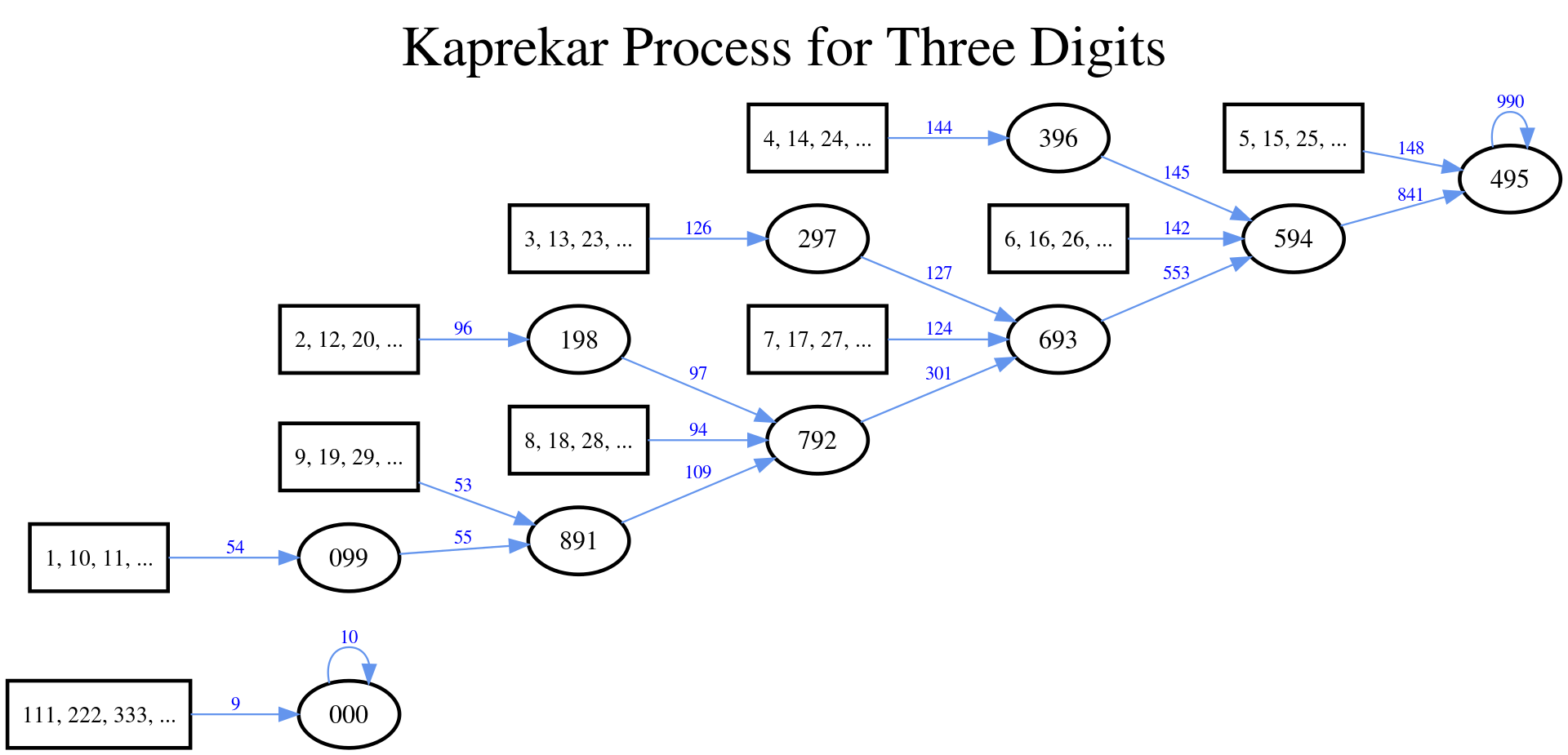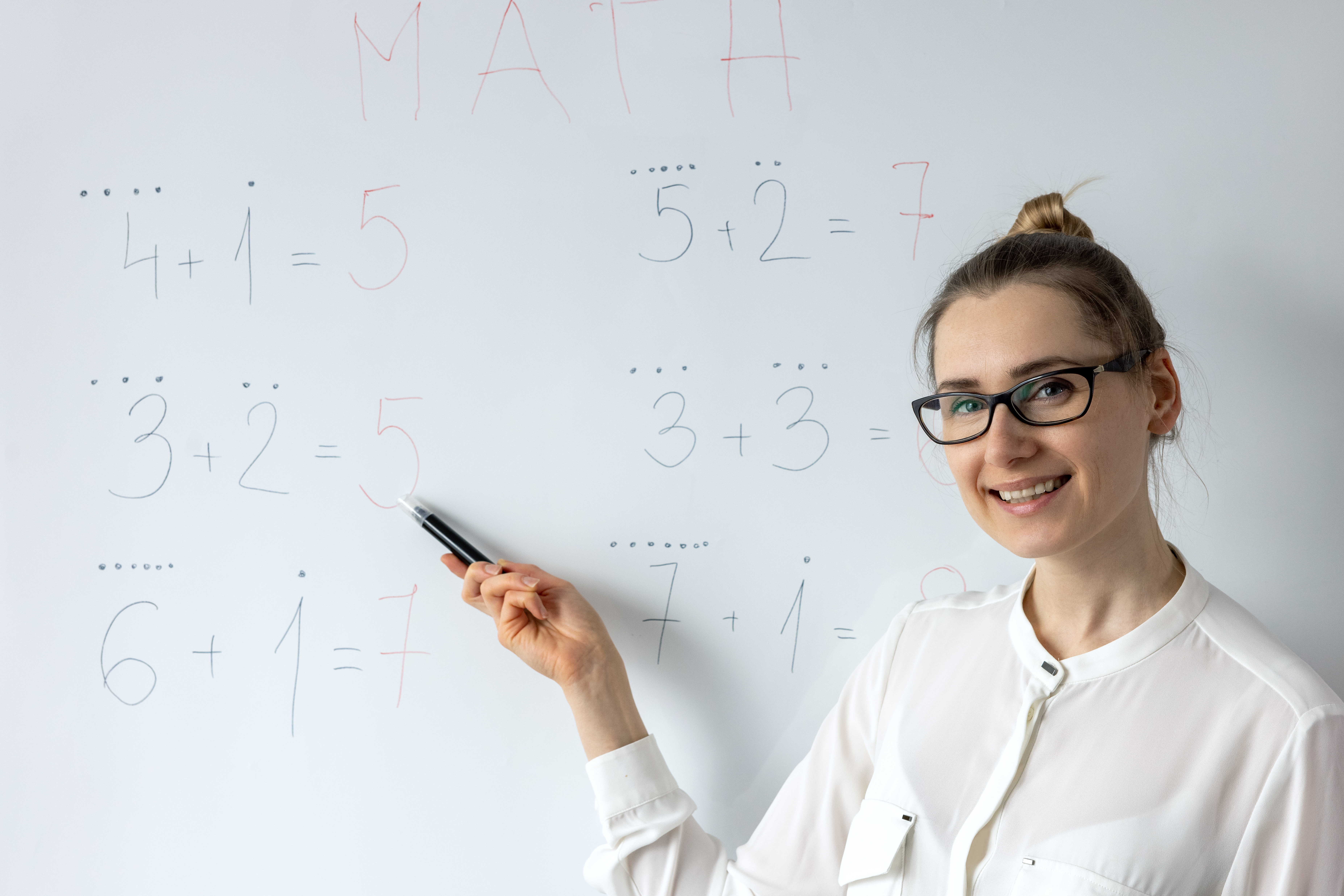Humans

# How To Ruin A TikTok Trick With Math

## It's a cool trick, but number theory is cooler.Published60Shares

TikTok, so often the source of life advice such as “how to accidentally inhale gases banned by the Geneva Convention” or “how to ruin a whole bunch of scientific studies,” has discovered number theory.

In a video posted Tuesday, user Math Letters showed off the special property of the number 495 – which he labels the three-digit Kaprekar constant.

Running through what mathematicians know as the Kaprekar routine, the narrator shows how any three-digit number – the only restriction is that you can’t choose all three digits to be the same, so no 111 or 222 or similar – will eventually end up at 495.

All you have to do is rearrange the digits from largest to smallest, then reverse that and take the difference between the two. Whatever you get, repeat the process. Within a maximum of six repetitions, you’ll get to 495 – and once you’re there, you’ll stay there.It’s a neat trick, and it certainly seems to work with any number we try. It’s also extremely generalizable: in fact, “the” Kaprekar number isn’t actually 495, but 6174, because D. R. Kaprekar, the Indian mathematician who first discovered the algorithm back in 1949, was originally working with four-digit numbers.

But let’s stick with three for now – is it always going to work? Are there any numbers that end up at a different number instead of 495? And how could we tell if they did?

Well, the good thing about math problems is that we can often prove them rigorously – and in this case, we have two options: to prove them by brains or brute force. And since the latter option involves running through 990 individual cases and checking whether they end up at 495, we’re going to be smart about it.

We happen to be working in base ten because that’s the default in the Western world. But to be clear, this proof works in any base you like – and that of course means you can find these constants in any base you like.

Step two: Pick an arbitrary number

Here’s a math tip: By “arbitrary”, we don’t mean “random”. We mean something like abc or xyz – something that can act as a stand-in for any three-digit number we like.

We’re also going to pull a little mathematical trick here, and say “wlog  b c.” In English, this is us saying that we are going to just assume the digits are already in descending order – if they aren’t, we can just rearrange it, so it doesn’t matter that we’re making this assumption.

Step three: Write your number as a sum

This is where the base comes in. Let’s say we chose abc – we know that a hundred and b-ty c can be written as a sum like this: 100a + 10b + c.

Step four: Reverse and repeat

If our starter number is abc, then in reverse order it’s cba. As a sum, this will be expressed as 100c + 10b + a.

Step five: Take the difference

So we have the larger number, 100a + 10b + c, and the smaller number, 100c + 10b + a, so if we take the difference we’re going to get:

(100a + 10b + c) – (100c + 10b + a) = 100(a – c) + (c – a) = 99(a – c)

Step six: What does this tell us?

What we just proved is that taking the difference between a large-to-small three-digit number and its reverse will always give us a multiple of 99. Which is true – you can try it – but it may seem a bit useless given what we’re trying to prove. But stick a pin in it – it will be important soon, we promise.

Let’s think back to what the question is: Is 495 the only non-zero number that is produced by the Kaprekar routine? Another way to phrase that would be: Is 495 the only non-zero number that produces itself under the Kaprekar routine?

That may seem like semantics, but it helps a lot in the proof, because now we have another bit of information about which numbers might be potential three-digit Kaprekar constants. Specifically, we know that if some permutation of abc is a constant, then it must be divisible by both 99 and (a – c).

Step seven: *Hand waving*

Using the same kinds of arguments as steps one through five, you can figure out that in base ten, (a – c) = 5.

Step eight: QED

So what have we found? If a three-digit number repeats itself under the Kaprekar routine, it can only be the product of 5 and 99. In other words, it must be 495. Hoorah! We made it!Now just to prove the same in all bases … and with four-digit numbers, five-digit numbers, and so on, and so on … you know what? Maybe we’ll just go back to those urban legends about 399-year-old Thai monks, it seems like less stress.

### ARTICLE POSTED INHumans
•• math,

• TikTok